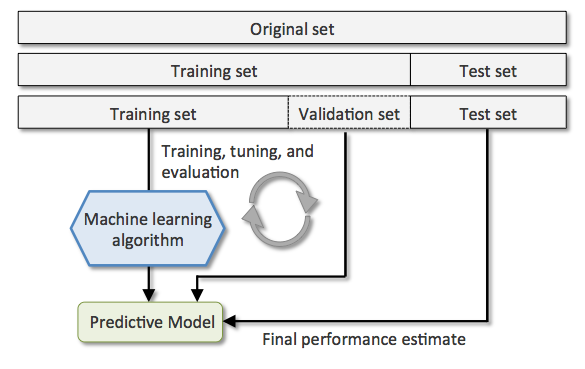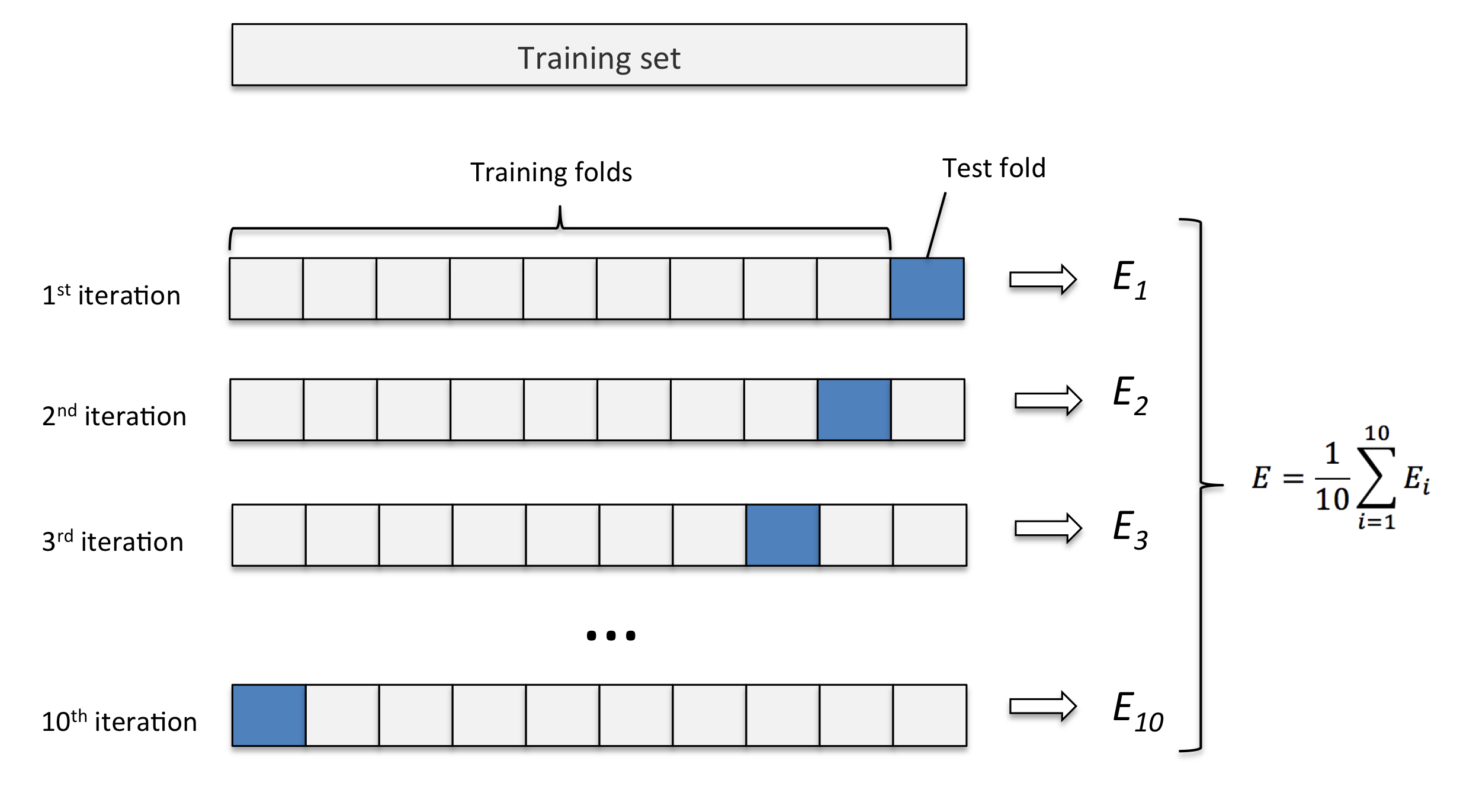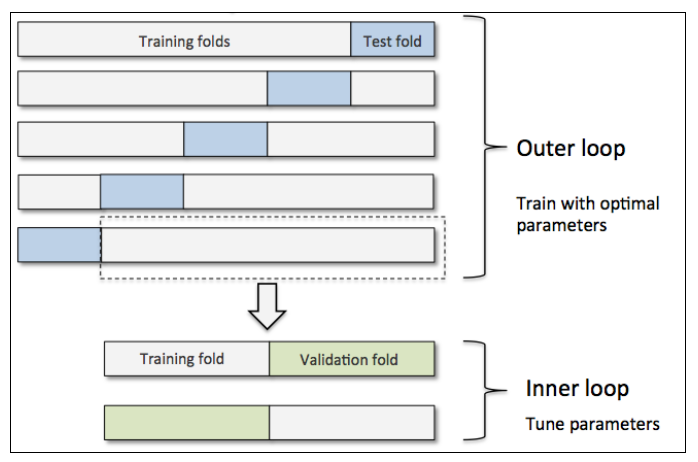# Cross Validation & Ensembling¶

Shan-Hung Wu & DataLab
Fall 2022

In this lab, we will guide you through the cross validation technique for hyperparameter selection. We will also practice the ensemble learning techniques that combine multiple base-leaners for better performance.

## Holdout Method¶

So far, we hold out the validation and testing sets for hyperparameter tuning and performance reporting. Specifically, we partition a dataset $\mathbb{X}$ into the training, validation, and testing sets. We use the training set to fit a model by giving a set of hyperparameters, and then use the validation set to evaluate the performance of the model given the hyperparameters. We repeat these two steps by issuing different sets of hyperparameters and pick the set that leads to the highest validation performance. We then use both the training and validation sets to train our final model, and apply it the testing set to evaluate/report the generalization performance. The following figure illustrates the procedure:Next, we apply this technique to evaluate the KNeighborsClassifier on the Iris dataset. For simplicity, we consider the sepal width and petal length features only. Let's split the dataset first:

Then, we iterate through each value of hyperparameter n_neighbors = 1, 15, 50 to train on the training set and estimate performance on the validation set and record the best:

One major disadvantage of the holdout method is that the validation and testing performance is sensitive to the random splits. If we have a unfortunate split such that the validation (resp. testing) set is unrepresentative, we may end up picking suboptimal hyperparameters (resp. reporting a misleading performance score).

In this case, the hyperparameter n_neighbors = 15 actually leads to better test performance:

We can see that the validation set is unrepresentative and leads to indistinguishable validation accuracy scores (1.0) for all values of n_neighbors.

Next, we take a look at a more robust technique called the $K$-Fold Cross-Validation.

## $K$-Fold Cross Validation¶

In $K$-fold cross-validation (CV), we randomly split the training dataset into $K$ folds without replacement, where $K−1$ folds are used for the model training and the remaining 1 fold is for testing. This procedure is repeated $K$ times so that we obtain $K$ models and $K$ performance estimates. Then we take their average as the final performance estimate. The following figure illustrate the 10-fold CV:We can apply $K$-fold CV to either the hyperparameter tuning, performance reporting, or both. The advantage of this approach is that the performance is less sensitive to unfortunate splits of data. In addition, it utilize data better since each example can be used for both training and validation/testing.

Let's use $K$-Fold CV to select the hyperparamter n_neighbors of the KNeighborsClassifier:

The dataset is first split into training/testing sets.

5-fold CV selects the best n_neighbors = 15 as we expected. Once selecting proper hyperparameter values, we retrain the model on the complete training set and obtain a final performance estimate on the test set:

### Nested CV¶

We can also apply the $K$-fold CV to both the hyperparameter selection and performance reporting at the same time, this is called the nested CV. Following illustrate the $5\times 2$ nested CV:where we select the values of hyperparameters by 2-fold CV and estimate the generalized performance by 5-fold CV, respectively. Let's try this ourselves:

As we can see, the 5 inner CVs may select different values for the hyperparameter n_neighbors. In this case, the 1st inner CV selects n_neighbors = 1 due to an unlucky split of the training and testing sets in the outer fold. By doing nested CV, we get a more robust performance estimation.

In fact, we can simplify the above example using the GridSearchCV from Scikit-learn:

NOTE: if we have a dataset with imbalance classes, we should use the stratified $K$-fold CV that prepserves the class proportions in each fold to ensure that each fold is representative of the class proportions in the training dataset. To use stratified CV, simply replace

>>> from sklearn.model_selection import KFold
>>> KFold(n_splits=...)

with

>>> from sklearn.model_selection import StratifiedKFold
>>> StratifiedKFold(y=..., n_splits=...)

### How Many Folds?¶

How many folds $K$ do we need? Here are some rules of thumb explained in the lecture:

• For large $K$, the MSE of cross-validation error (to the true expected generalization error of $f_N$) tends to have a small bias but large variance since a classifier in each iteration is trained on more examples but classifiers from different folds are trained on similar examples;
• On the other hand, for small $K$, the cross-validation error tends to have large bias but small variance;
• When dataset is small, the cross-validation error will have both large bias and large variance.

To see these in practice, let's consider the Polynomial regression where the ground truth data generating distribution is known:

$$\mathrm{P}(\mathrm{y}|\mathrm{x}) = \sin(x) + \epsilon, \epsilon\sim\mathcal{N}(0,\sigma^2)$$

We can visualize the bias and variance as follows:

Usually, we set $K=10$ in most applications, $K=5$ for larger datasets, and $K=N$ for very small datasets. The last setting is called the leave-one-out CV.

## Ensemble Methods¶

No free lunch theorem states that no machine learning algorithm is universally better than others in all domains. The goal of ensembling is to combine multiple learners to improve the applicability and get better performance.

NOTE: it is possible that the final model performs no better than the most accurate learner in the ensemble models. But it at least reduces the probability of selecting a poor one and increases the applicability.

### Voting¶

Voting is arguably the most straightforward way to combine multiple learners $d^{(j)}(\cdot)$. The idea is to taking a linear combination of the predictions made by the learners. For example, in multiclass classification, we have

$$\tilde{y}_k =\sum_j^L w_j d^{(j)}_k(\boldsymbol{x}), \text{ where }w_j\geq 0\text{ and }\sum_j w_j=1,$$

for any class $k$, where $L$ is the number of voters. This can be simplified to the plurarity vote where each voter has the same weight:

$$\tilde{y}_k =\sum_j \frac{1}{L} d^{(j)}_k(\boldsymbol{x}).$$

Let's use the VotingClassifier from Scikit-learn to combine KNeighborsClassifer, LogisticRegression, and DecisionTreeClassifier together and train on the synthetic two-moon dataset: